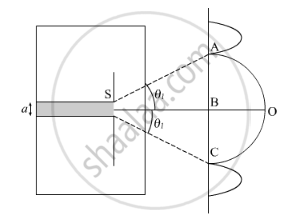# Show that the Angular Width of the First Diffraction Fringe is Half that of the Central Fringe. - Physics

Show that the angular width of the first diffraction fringe is half that of the central fringe.

#### SolutionLet λ and a be the wavelength and slit width of diffracting system, respectively. Let O be the position of central maximum.  Condition for the first minimum is given by

asinθ=mλ     .....(1)

Let θ be the angle of diffraction.

As diffraction angle is small

sinθ ≈ θ

For the first diffraction minimum, let θ = θ1

For the first minimum, take m =1

aθ1=λ

=>theta_1=lambda/a

Now, angular width AB = θ1

Angular width BC = θ1

Angular width AC = 2θ1

Width of central maximum = 2θ1

Concept: Interference of Light Waves and Young’S Experiment
Is there an error in this question or solution?